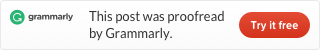# LeetCode problem 23 Merge k Sorted Lists [Divide and conquer][Min-Heap]（Java）

Merge k sorted linked lists and return it as one sorted list. Analyze and describe its complexity.

Example:

Input:
[
1->4->5,
1->3->4,
2->6
]
Output:
1->1->2->3->4->4->5->6

I don’t know how to merge k lists, but we know how to merge two lists from problem 21. So we can merge the first two lists and merge others one by one. This is the brute force solution.

## Brute force solution

mergeTwoList function from problem 21.

## Divide and conquer solution

How to use Divide and conquer approach to solve this problem? We can naturally think about divide the lists to two half, then divide it again and again, until there are only two linked lists. Then we can use the mergeTwoList function from problem 21. And we return to combine the results. So this is just like Mergesort from book Introduction to Algorithms, and we already know how to write. Our solution is almost identical to our Mergesort code.

## Min-heap solution

In this solution we use a min-heap, it is almost identical to max-heap. We only need to change serval lines to make a max-heap to become min-heap. We use the code described in “Heap and Heap-Sort algorithm“.

Our method is: First, move all data from lists to an array, then use the array to build a min-heap. Finally, we output the current minimum number in a loop, make a LinkedList and return it.

This is the code (Code about min-heap you can find in GitHub files):